# Problems of the surface area

#### Number of problems found: 481

• GreenhouseThe Garden plastic greenhouse is shaped half-cylinder with a diameter of 6 m and base length 20 m. At least how many m2 of plastic is needed for its cover?
• Tent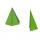Pyramid-shaped tent has a base square with a side length of 2 m and a height 1.7 m. How many meters of canvas is nneded to make it if for a waste should be added 10%?
• Sphere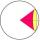Intersect between plane and a sphere is a circle with a radius of 60 mm. Cone whose base is this circle and whose apex is at the center of the sphere has a height of 34 mm. Calculate the surface area and volume of a sphere.
• Triangular pyramidIt is given perpendicular regular triangular pyramid: base side a = 5 cm, height v = 8 cm, volume V = 28.8 cm3. What is it content (surface area)?
• Cylinder diameter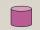The surface of the cylinder is 149 cm2. The cylinder height is 6 cm. What is the diameter of this cylinder?
• Iron sphereIron sphere has weight 100 kg and density ρ = 7600 kg/m3. Calculate the volume, surface, and diameter of the sphere.
• Spherical cap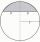What is the surface area of a spherical cap, the base diameter 20 m, height 2 m.
• Triangular prism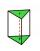The base perpendicular triangular prism is a right triangle whose hypotenuse measures 5 cm and one cathetus 2 cm. Height of the prism is equal to 7/9 of the perimeter of the base. Calculate the surface area of prism.
• Sphere - partsCalculate the area of a spherical cap, which is part of an area with base radius ρ = 9 cm and a height v = 3.1 cm.
• The body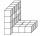The body on the figure consists of cubes with an edge length 10 cm. What surface has this body?
• PitThe pit has the shape of a truncated pyramid with a rectangular base and is 0.8 m deep. The pit's length and width are the top 3 × 1.5 m bottom 1 m × 0.5 m. To paint one square meter of the pit we use 0.6 l of green color. How many liters of paint are nee
• Mystery of stereometrieTwo regular tetrahedrons have surfaces 88 cm2 and 198 cm2. In what ratio is their volumes? Write as a fraction and as a decimal rounded to 4 decimal places.
• Cone and the ratioThe rotational cone has a height 43 cm, and the ratio of the base surface to lateral surface is 5: 7. Calculate the surface of the base and the lateral surface.
• SailsWe known heights 220, 165, and 132 of sail. It has a triangular shape. What is the surface of the sail?
• BoxCardboard box-shaped quadrangular prism with a rhombic base. Rhombus has a side 5 cm, and one diagonal 8 cm long, and the box's height is 12 cm. The box will open at the top. How many cm2 of cardboard do we need to cover overlap and joints that are 5% of
• CD disc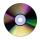A compact disc has a diameter of 11.8 cm. What is the surface area of the disc in square centimeters?
• Funnel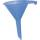The funnel has the shape of an equilateral cone. Calculate the surface wetted with water if we poured into the funnel 7.1 liters of water.
• Prism XThe prism with the edges of the lengths x cm, 2x cm, and 3x cm has volume 20250 cm3. What is the area of the surface of the prism?
• TowerThe top of the tower is a regular hexagonal pyramid with base edge 8 meters long and a height 5 meters. How many m2 of the sheet is required to cover the top of the tower if we count 8% of the sheet waste?
• Cuboid diagonalCalculate the volume and surface area of the cuboid ABCDEFGH, which sides a, b, c has dimensions in the ratio of 9:3:8. If you know that the diagonal wall AC is 86 cm, and the angle between AC and space diagonal AG is 25 degrees.

Do you have an exciting math question or word problem that you can't solve? Ask a question or post a math problem, and we can try to solve it.

We will send a solution to your e-mail address. Solved examples are also published here. Please enter the e-mail correctly and check whether you don't have a full mailbox.